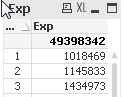# QlikView Scripting

Discussion Board for collaboration on QlikView Scripting.

Highlighted
Contributor

## Sum over aggr (in text object)

Dear Community,

i have a straight table with one dimension 'Group' and one expression '=SUM(Aggr(SUM(AMOUNT),GROUP))'

On the top are the totals by expression

The result looks like this :Now i want to display the total 49398... directly inside a text object as a key performance indicator using the same expression

=SUM(Aggr(SUM(AMOUNT),GROUP)). But its not working as the number is not the same comparing with the straight table totalTags (3)
1 Solution

Accepted Solutions
HighlightedMVP

## Re: Sum over aggr (in text object)

maybe this

=sum({\$ <Group*={"*"}>} Amount)

14 Replies
HighlightedMVP

## Re: Sum over aggr

Aggr works with the dimensions inside the chart so try to add all the dimensions used in the chart to your aggr statement

HighlightedPartner

## Re: Sum over aggr

you need to ignore dimension by using total

ex:sum(total column)

I can walk on water when it freezes
Highlighted
Contributor

## Re: Sum over aggr (in text object)

Inside the straight table we have only one dimension called 'Group', so the total value should be the same inside the table and text object

Highlighted
Contributor

## Re: Sum over aggr (in text object)

I tried to use =SUM(Aggr(SUM(Total AMOUNT),GROUP)) but the value was also wrong

HighlightedMVP

## Re: Sum over aggr (in text object)

Could you please try with the following expession in the chart and in the text box

'=SUM(Aggr(NODISTINCT SUM(AMOUNT),GROUP))'

HighlightedPartner

## Re: Sum over aggr (in text object)

may you please supply a sample QVW ?

I can walk on water when it freezes
HighlightedMVP

## Re: Sum over aggr (in text object)

This expression in chart with Group as a dimension

=SUM(Aggr(SUM(AMOUNT),GROUP))

is just an inefficient way of saying

=SUM(AMOUNT)

The first expression is the correct way of expressing the sum and is the equivalent of the chart. If the totals are different, then you are using an expression total rather than a sum of rows, and the equivalent would then be the second expression. Unless you have some restrictions in the chart such as a calculated dimension that filters GROUP.

If that does not resolve the problem, then I suggest you post your qvw or a representative sample to see why it is behaving this way.

Logic will get you from a to b. Imagination will take you everywhere. - A Einstein
Highlighted
Contributor

## Re: Sum over aggr (in text object)

I have prepared an example qvw.file please see attached.

Highlighted
Contributor

## Re: Sum over aggr (in text object)

See attachement below